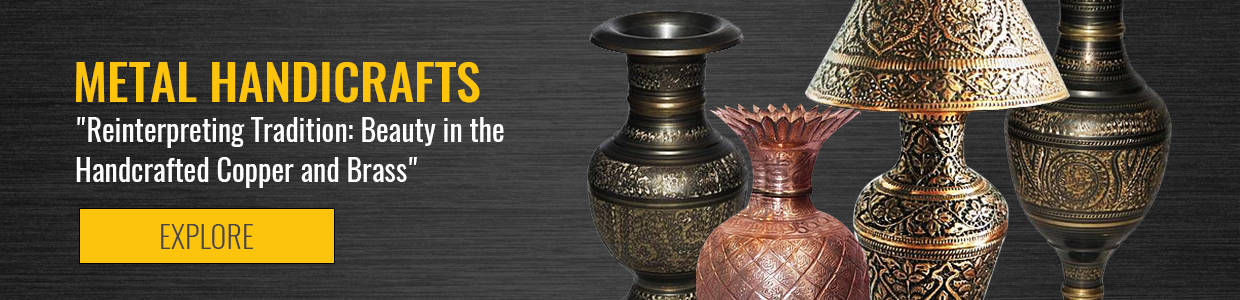CURRENCY## Metal Crafts

Brass and copper have been worked in the northern Pakistan lands ever since the Mughals invaded and were amazed by the skill of local artisans. Extremely intricate carving on brass and metal is done in Swat and old bazaars of Peshawar to create wondrous creations which can momentarily stun you with their craftsmanship.

.

X

View Filter

x
Clear all x

Rs. 1,650
Rs. 1,650
Rs. 1,650
Rs. 3,300
Rs. 1,650
Rs. 1,650
Rs. 610
Rs. 610
Rs. 620
Rs. 940
Rs. 6,600
Rs. 6,270
Rs. 6,270
Rs. 6,270
Rs. 6,270
Rs. 1,540
Rs. 7,430
Rs. 7,430
Rs. 2,810
Rs. 2,810
Rs. 1,820
Rs. 1,820
Rs. 1,980
Rs. 990
Rs. 990
Rs. 1,410
Rs. 1,410
Rs. 4,620
Rs. 6,930
Rs. 590
Rs. 590
Rs. 1,490
Rs. 1,490
Rs. 770
Rs. 940
Rs. 1,820
Rs. 660
Rs. 660
Rs. 660
Rs. 660
Rs. 660
Rs. 1,980
Rs. 1,820
Rs. 1,980
Rs. 1,980
Rs. 1,820
Rs. 2,320
Rs. 2,320
Rs. 2,970
Rs. 1,980
Rs. 2,970
Rs. 1,980
Rs. 3,960
Rs. 4,400
Rs. 6,930
Rs. 6,930
Rs. 6,930
Rs. 4,760
Rs. 8,000
Rs. 7,500
Rs. 9,950
Rs. 9,950
Rs. 7,000
Rs. 1,500
Rs. 2,000
Rs. 2,300
Rs. 1,000
Rs. 4,500
Rs. 4,500
Rs. 3,500
Rs. 3,500
Rs. 3,500
Rs. 9,000
Rs. 1,200
Rs. 1,200
Rs. 1,200
Rs. 1,200
Rs. 1,600
Rs. 1,600
Rs. 1,450
Rs. 950
Rs. 1,450
Rs. 2,850
Rs. 2,850
Rs. 350
Rs. 350
Rs. 350
Rs. 350
Rs. 350
Rs. 3,200
Rs. 4,000
Rs. 955
Rs. 600
Rs. 400
Rs. 500
Rs. 700
Rs. 1,500
Rs. 700
Rs. 700
Rs. 1,500